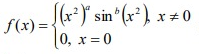# What must a and b be for the function to be continuous on R?

### What must a and b be for the function to be continuous on R?What must a and b be for the function to be continuous on R?
Guest

### Re: What must a and b be for the function to be continuous o

First it should be obvious that is a and b are both positive then the limit is 0 so the function is continuous. I would let $$u= x^2$$ so the limit becomes $$\lim_{u\to 0}u^a sin^b(u)$$. Because $$\lim_{x\to 0} \frac{sin(x)}{x}= 1$$ I would then rewrite it as $$\lim_{x\to 0} u^{a- b}\left(\frac{sin(u)}{u}\right)^b$$. It looks to me like $$\lim_{x\to 0} \left(\frac{sin(u)}{u}\right)^b= 1$$ for any b so we must have $$\lim_{u\to 0} u^{a- b}= 0$$ and that requires that a> b.

HallsofIvy

Posts: 308
Joined: Sat Mar 02, 2019 9:45 am
Reputation: 107